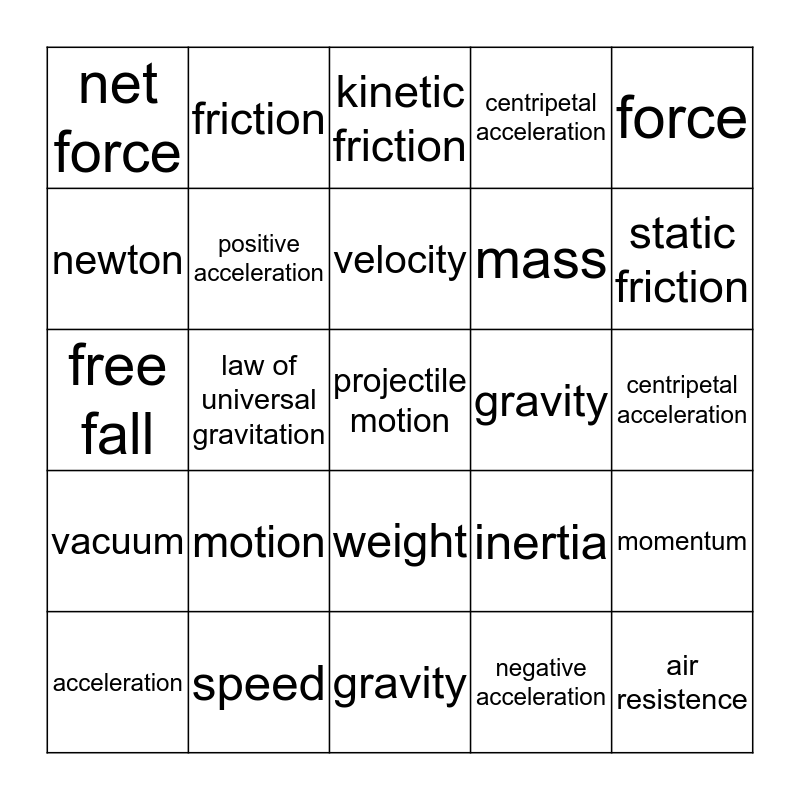# Unit 5: Motion, Forces, and WorkThis bingo card has a free space and 24 words: motion, speed, velocity, acceleration, force, net force, newton, friction, gravity, weight, mass, free fall, inertia, momentum, positive acceleration, negative acceleration, centripetal acceleration, kinetic friction, static friction, law of universal gravitation, air resistence, vacuum, centripetal acceleration and gravity.

⚠ This card has duplicate items: gravity (2), centripetal acceleration (2)

## Play Online